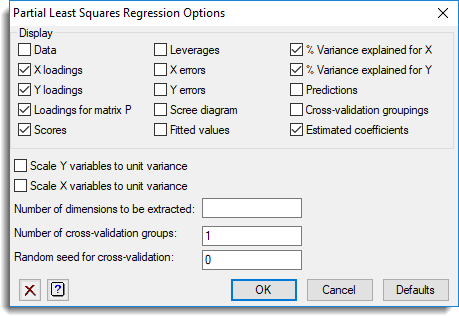1. Home
2. Partial Least Squares Options

# Partial Least Squares Options

Use this to select options and output to be used by partial least squares regression.## Display

Specifies which items of output are to be displayed in the Output window.

## Scale Y variables to unit variance

Controls whether to scale the Y variates to unit variance

## Scale X variables to unit variance

Controls whether to scale the X variates to unit variance

## Number of dimensions to be extracted

Specified the number of dimensions to be extracted

## Number of cross-validation groups

Specifies the number of cross-validation groups into which to divide the data. If you do not want cross-validation then leave this blank or enter 1.

## Random seed for cross-validation

Specifies a random seed value to use when dividing the data randomly into the cross-validation groups.

Updated on April 25, 2019